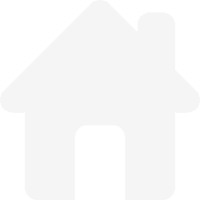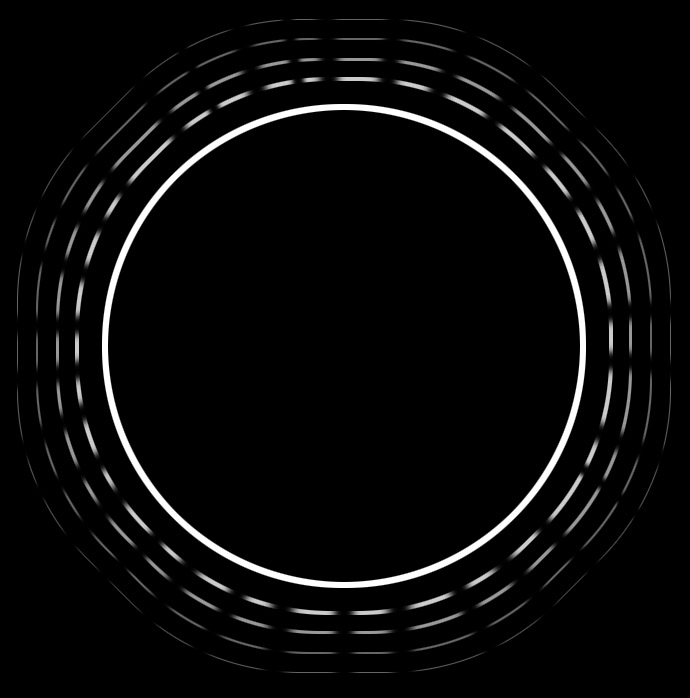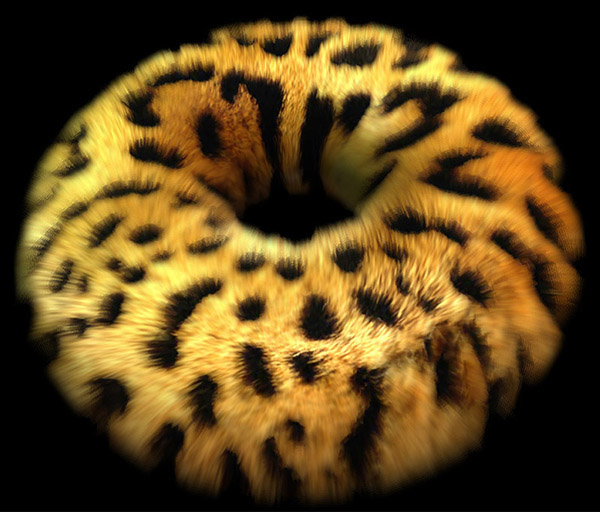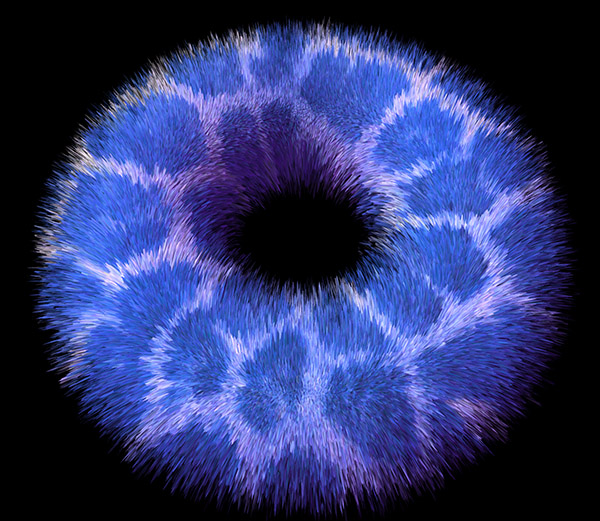# Jim's GameDev Blog# 皮毛渲染

2016-8-12``````// vertex
v.vertex.xyz += v.normal * _FurLength * FUR_DEPTH;

// fragment
half4 c = tex2D (_MainTex, IN.uv_MainTex);
o.Albedo = c.rgb * _Color.rgb;
half alpha = step(lerp(_Cutoff, _CutoffEnd, FUR_LAYER), c.a);
o.Alpha = alpha * (1 - FUR_LAYER);
``````

FUR_LAYER 表示当前是第几层。通过 _MainTex 的 Alpha 通道中的信息来控制皮毛的稀疏和长短。每一层都需要重复执行以上的着色器，只是 FUR_LAYER 会有所差异。

``````// Fur Pass 1
CGPROGRAM
#pragma surface surf BlinnPhong vertex:vert alpha
#define FUR_MULTIPLIER 0.05
#include "MyFurInclude.cginc"
ENDCG

// Fur Pass 2
CGPROGRAM
#pragma surface surf BlinnPhong vertex:vert alpha
#define FUR_MULTIPLIER 0.10
#include "MyFurInclude.cginc"
ENDCG

// Other Passes
````````````#pragma surface surf BlinnPhong vertex:vert tessellate:tessEdge alpha:blend

float4 tessEdge(appdata v0, appdata v1, appdata v2)
{
return UnityEdgeLengthBasedTess(v0.vertex, v1.vertex, v2.vertex, _EdgeLength);
}

void vert(inout appdata v)
{
float4 dispC = tex2Dlod(_DispTex, float4(v.texcoord.xy, 0, 0));
float d = dispC.r * _Displacement;
v.vertex.xyz += v.normal * d - dispC.g * v.normal * _Displacement2;
}
``````